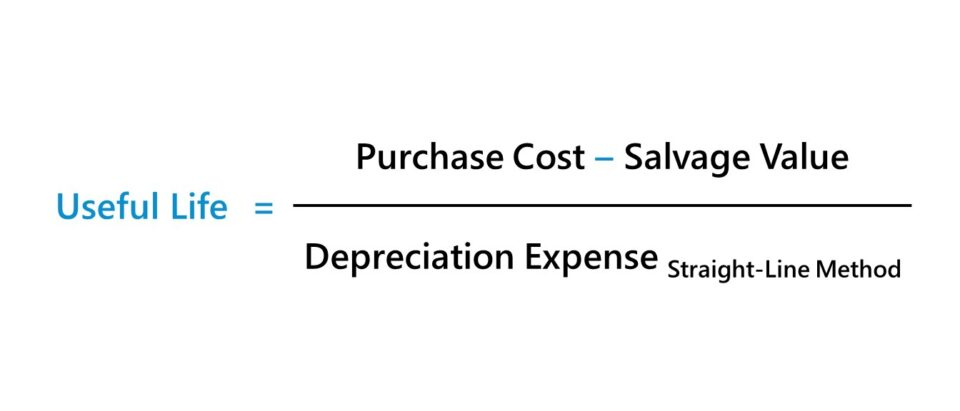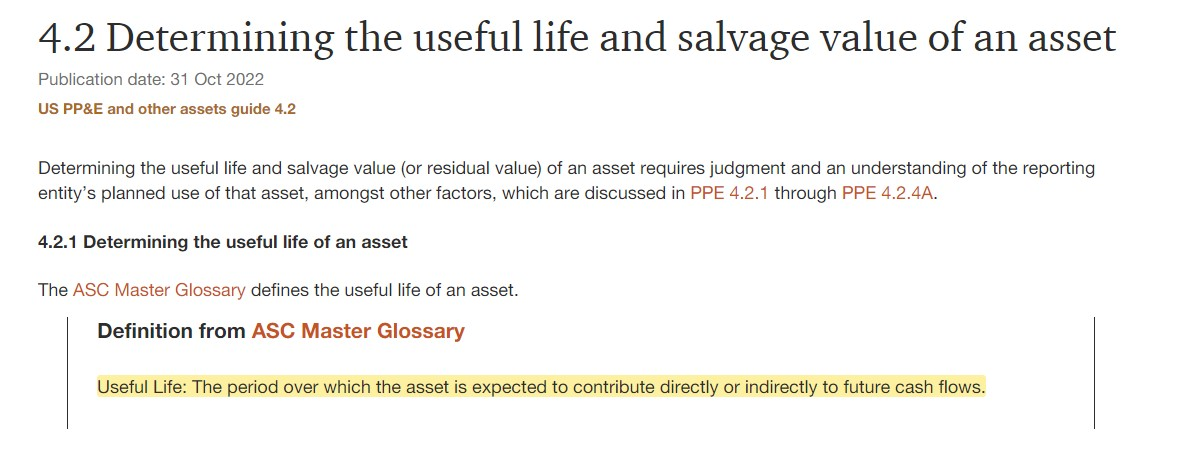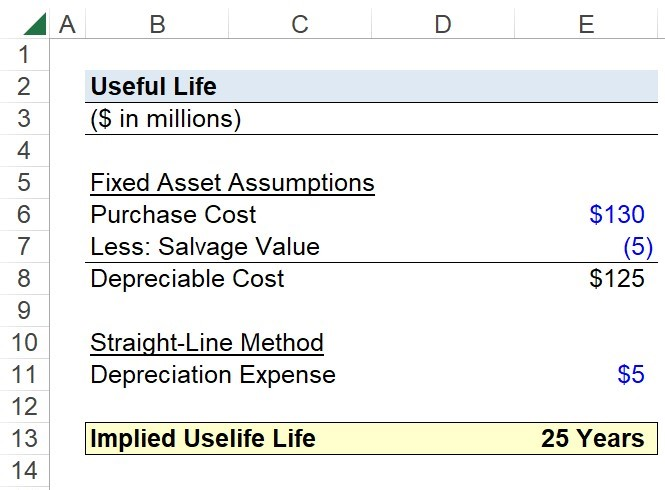Welcome to Wall Street Prep! Use code at checkout for 15% off.# Useful Life

Guide to Understanding the Useful Life Assumption## How to Calculate Useful Life (Step-by-Step)

An asset’s useful life assumption refers to the estimated number of years that the company’s management team expects it to be able to contribute positive economic value.

The useful life concept is only applicable to non-current fixed assets and intangible assets as part of accrual accounting reporting standards, where an assumption regarding the estimated useful life is required on the date of purchase (or acquisition).

• Fixed Asset: The tangible resources belonging to a company expected to provide economic benefits over the long term, i.e. the benefits are not fully realized for a period in excess of twelve months. For example, the purchase of machinery is anticipated to provide utility for more than one year, while a current asset like inventory is expected to cycle out within twelve months.
• Intangible Asset: The non-physical assets of a company, which can include patents, copyrights, trademarks, and intellectual property (IP). Unlike fixed assets, the value of an intangible asset (or the useful life assumption) is not recognized on the books until certain events such as becoming the target of an acquisition.

Some common examples of fixed assets that must be depreciated are the following:

• Property, Plant and Equipment (PP&E)
• Factories
• Machinery and Tools
• Transportation Vehicles (e.g. Cars, Trucks)

The cost of purchasing fixed assets (i.e. PP&E) must be recognized on the income statement through depreciation expense, in an effort to allocate the original purchase price (i.e. the capital expenditure) across the asset’s estimated useful life.

The rationale behind the conception of depreciation (and amortization for intangible assets) is tied to the matching principle of accrual accounting, where the expense is recorded in the same period as when the corresponding benefit was derived.

Not all non-current fixed and intangible assets are depreciated or amortized, however, such as land and goodwill.

• Land: Land is assumed to have an indefinite useful life.
• Goodwill: Goodwill is tested for annual impairment for publicly-traded companies.“Determining the Useful Life and Salvage Value of an Asset” (Source: PWC)

## Useful Life Formula

The calculation of the implied useful life of an asset is uncommon, i.e. the accounting methodology and assumptions can typically be found in the financial filings (10-K or 10-Q).

However, the implied useful life can be determined by rearranging the formula for calculating depreciation expense.

Useful Life = (Purchase Cost Salvage Value) ÷ Depreciation Expense
• Purchase Cost: The cost of purchasing the non-current asset, i.e. the entire capital expenditure (Capex) amount.
• Salvage Value: The residual value of the asset remaining at the end of the asset’s useful life, i.e. the “scrap” value that it could be sold for in the market.
• Depreciation: The annual depreciation expense recognized on the income statement in each period.

Note: The useful life formula assumes the straight-line depreciation method is used, in which the depreciation expense is recorded in equal installments.

## Understanding Useful Life Assumption vs. Depreciation Expense

The general rules for interpreting the relationship between the recorded depreciation expense and the useful life assumption are straightforward.

• Longer Useful Life Assumption → Lower Depreciation Expense (and Higher Net Income + EPS)
• Shorter Useful Life Assumption → Higher Depreciation Expense (and Lower Net Income + EPS)

The total depreciation expense remains the same, regardless of the useful life assumption or the depreciation method.

But considering the near-term focus of public companies, where management teams and financial performance are evaluated based on their earnings guidance and external EPS targets set by equity analysts, companies have the incentive to minimize the depreciation expense.

Hence, most companies use the straight-line depreciation method to show higher earnings, as opposed to accelerated depreciation for purposes of bookkeeping, which tends to benefit their share price in the near term.

## Useful Life Calculator — Excel Template

We’ll now move on to a modeling exercise, which you can access by filling out the form below.Submitting...

## Step 1. Fixed Asset Assumptions (PP&E)

Suppose you’re tasked with determining the useful life assumption of a fixed asset that a manufacturer purchased using the following financial assumptions.

Fixed Asset Assumptions (PP&E)

• Purchase Cost = \$130 million
• Salvage Value = \$5 million
• Depreciation Expense = \$5 million (Straight-Line Method)

The incurred capital expenditure (Capex) amounted to \$130 million, i.e. the total cost of purchasing the fixed asset (PP&E), while the residual value left over at the end of its useful life is \$5 million. Thus, the depreciable cost of the fixed asset is \$125 million.

• Depreciable Cost = \$130 million – \$5 million = \$125 million

## Step 2. Useful Life Calculation Example

Under the straight-line method, the annual depreciation expense is \$5 million, so we can divide our depreciable cost by the depreciation expense to determine the implied useful life assumption by management.

• Useful Life = \$125 million ÷ \$5 million = 25 Years

In closing, the implied useful life assumption of the fixed asset is 25 years, so the \$5 million in depreciation is recognized on the income statement as an annual expense for a period of 25 years.Step-by-Step Online Course

### Everything You Need To Master Financial Modeling

Enroll in The Premium Package: Learn Financial Statement Modeling, DCF, M&A, LBO and Comps. The same training program used at top investment banks.